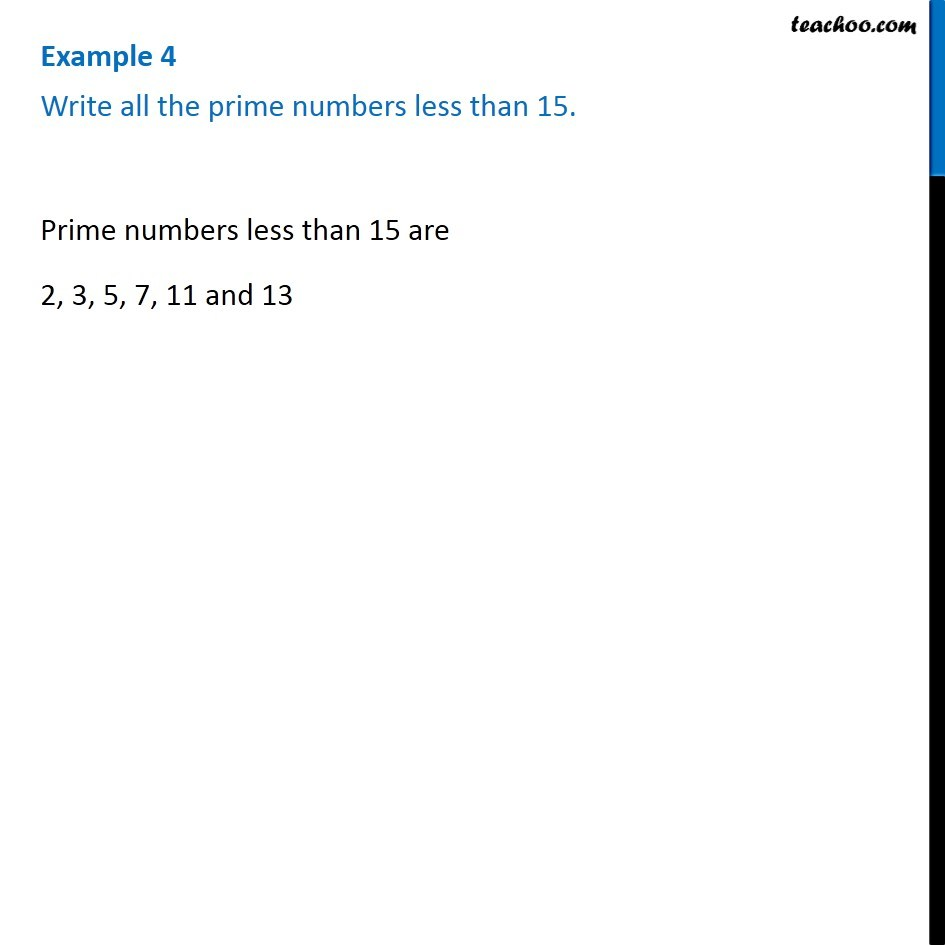1. Chapter 3 Class 6 Playing with Numbers
2. Serial order wise
3. Examples

Transcript

Example 4 Write all the prime numbers less than 15. Prime numbers are numbers with only two factors - 1 and the number itself Prime numbers less than 15 are 2, 3, 5, 7, 11 and 13

Examples